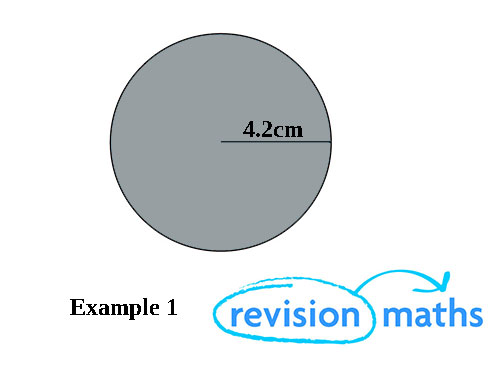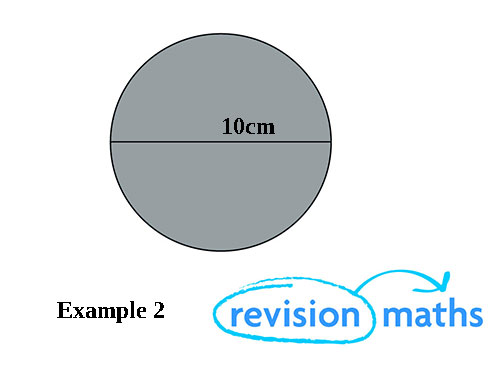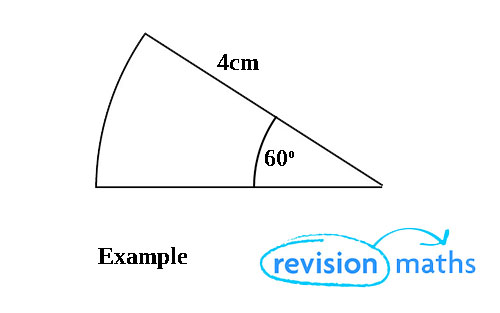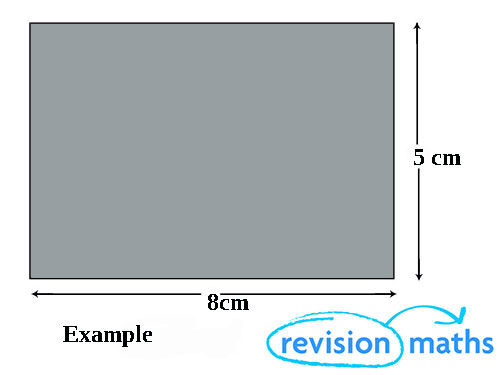## Perimeter

A Perimeter is a one dimensional measurement. It is a length measured usually in metric units such as CM M KM.

When calculating the perimeter of a shape, remember that you are looking for a measurement all the way around its edge. So simply add up all the sides of any shape. You must also remember all units of measurement are the same e.g. CM.

Perimeter of a Circle

The perimeter of a circle is known as the circumference. To calculate the circumference of a circle you can use one of two formulae, either: C = 2πr or C = πd. In these formulae r is the radius and d is the diameter.

Looking at example 1 below you can calculate the circumference as follows:C = 2 X π X r (6.28318 x 4.2) = 26.39 (to 4 significant figures)

Looking at example to below you can calculate the circumference as follows:C =  π X d (3.14159 x 10) = 31.42 (to 4 s. f.)

To calculate the perimeter of an arc (part of a circle) from the angle you can calculate the fraction of the circle.In the example above we can work this out as follows:

60/360 x 2π x 4 = 4/3 π cm

= 4.19cm (to 3 s.f.) The perimeter of the whole sector is therefore 4.19 + 8 = 12.19 (4 s.f.).

The perimeter of a rectangle

To calculate the perimeter of a rectangle, just add all the sides together.In the example above the answer is 26cm.